This is the dw-nominate-detail notebook.
If want less documentation, please view the dw-nominate notebook.

## DW-Nominate Exploration¶

The Nominate scoring scale was first developed by Keith T. Poole and Howard Rosenthal in the late 1980's.
Since then, it has undergone several iterations, with the DW series being the latest.
Scores are derived from roll call votes, anc contain 2 dimensions:

1. Allowing us to place Senators, House members, and their political orgs on the liberal-convervative [-1, 1] spectrum (1st dimension).
2. Nominate also quantifies the opposition/support of civil rights for underrepresented minorities (2nd dimension).

The following notebook is going to build off a visualization made by the Pew Research Institute, by making a gif of changes of house ideology accross congresses.

Here's the finished product:GIF hosted on Github.

We're going to

1. Read a fixed-width text file from an anonymous ftp hosted on the web.
2. Use Pandas dataframes to filter, replace, and aggregate data.
3. Plot data using Panda's Matplotlib extension.
4. Generate a GIF out of static png files.

We're going to use the following open sourced modules.

In [ ]:
# this is for displaying graphs within the Notebook
%matplotlib inline

In :
import os           # operating system, used for file manipulation
import glob         # used to list files with a Regular Epression (RegEx)

import us           # translates state names to codes
import numpy as np  # linear algebra, matrix manipulation
import pandas as pd # data wrangling and analysis suite
import matplotlib.pyplot as plt    # plotting
import matplotlib.ticker as ticker # plot styling
import imageio      # for making animations


We can access files hosted on an anaymous File Transfer Protocol (FTP), as if it were a local file. Below are variables for where the raw files are hosted:

In :
senate_dl = 'ftp://k7moa.com/junkord/SL01113D21_BSSE.dat'
house_dl = 'ftp://k7moa.com/junkord/HL01113D21_BSSE.DAT'


We can store into of the senate and house of representatives as a list of tuples.

In :
args = [('senate', senate_dl),
('house', house_dl)]


Lists can hold any dtype, we can access the first element using an index by a variable within two hard brackets.

In :
args

Out:
('senate', 'ftp://k7moa.com/junkord/SL01113D21_BSSE.dat')

Because the tuple is also list-like, we can index the last variable.

In :
args[-1]

Out:
'ftp://k7moa.com/junkord/SL01113D21_BSSE.dat'
In :
for arg in args:
print(arg)

ftp://k7moa.com/junkord/SL01113D21_BSSE.dat
ftp://k7moa.com/junkord/HL01113D21_BSSE.DAT


columns copied and pasted from voteview docs, and parsed using split.

In :
cols = '''Congress Number
ICPSR ID Number
State Code
Congressional District Number
State Name
Party Code
Name
1st Dimension Coordinate
2nd Dimension Coordinate
1st Dimension Bootstrapped Standard Error
2nd Dimension Bootstrapped Standard Error
Correlation Between 1st and 2nd Dimension
Log-Likelihood
Number of Classification Errors
Geometric Mean Probability'''.split('\n')

In :
"We split the columns by a newline character (\n), to get {} columns.".format(len(cols))

Out:
'We split the columns by a newline character (\n), to get 16 columns.'
In :
cols

Out:
'Congress Number'

Below is a list of tuples, containig the characterspaces between each column above.

In :
col_widths = [(0,4), (4,10), (10,13), (13,15),
(15,23), (23,28), (28,40), (40,50),
(50,60), (60,70), (70,80), (80,90),
(90,102), (102,107), (107, 112)]

In :
# This dict is for compat. with Propublica Congress API.
col_mapping = {'Name' : 'last_name',
'Congress Number': 'Congress'}

# dict comprehension to convert state names to abbreviations.
state_dict = {state.name.upper()[:7] : state.abbr for state in us.states.STATES}


In :
df = pd.read_fwf(house_dl, names=cols, colspecs=col_widths)


Let's read both files into one dataframe. We're then going to replace column names using the col_mapping dictionary, and a list comprehension. Lastly, we're creating two columns--

1. for senate or house and

2. for the state code (replaced incomplete state name).

In :
df_congress = pd.DataFrame()

for chamber in args:

# replace column names from col_mapping dict
df.columns = [col_mapping.get(col, col) for col in df.columns]

# create column for senate or house
df['chamber'] = chamber

# convert state names to state id.
df['state'] = df['State Name'].replace(state_dict)

df_congress = df_congress.append(df, ignore_index=True)


Let's check we processed both senate and house

In :
df_congress.chamber.unique()

Out:
array(['senate', 'house'], dtype=object)

let's set some variables for the next graph.

In :
first_congress_yr = 1789
colors = ['b', 'r']
annotation = ('Ideology score from 1st Dimensional Coordinate of DW-Nominate\n'
'Source: voteview.com/dwnomin.htm\nAuthor: @leonyin')
col_name = 'More Liberal' + (57 * ' ') + 'More Conservative'

# these are preset values for the house and senate graphs.
house_plot = {
'ylim' : [0, 80],
'y_maj' : 20,
'y_min' : 10,
'ylabel': '# House Reps'
}

senate_plot ={
'ylim' : [0, 24],
'y_maj' : 8,
'y_min' : 4,
'ylabel' : '# Senators'
}


Next we define two if/else functions to create a nominal modified to the congress number and convert Party Code into a Party Name.

In :
def get_nominal(x):
'''
Returns a nominal for each congress number
'''
if x % 10 == 1 and x < 110:
return 'st'
elif x % 10 == 2 and x < 110:
return 'nd'
elif x % 10 == 3 and x < 110:
return 'rd'
else:
return 'th'

def rep_dem_indie(row):
'''
Maps Democrat, Republican, or Other for a given Pandas row.
'''
if row['Party Code'] == 100:
return 'D'
elif row['Party Code'] == 200:
return 'R'
else:
return None

In :
def plot_polarity(congress, chamber='senate', how='area',
col='1st Dimension Coordinate'):
'''
Plot the liberal-conservative polarity for a congress
for either house of reps or senate.

The default metric plotted is the 1st dim coordinate.
'''
if chamber == 'house':
plot_vars = house_plot
else:
plot_vars = senate_plot

# Set variables for title
congress_year = first_congress_yr + (2 * congress) - 2
congress_nominal = str(congress) + get_nominal(congress)

# create supertitle and subtitle.
sup_title = '{} Ideology'.format(chamber.title())
title = '{} Congress {}-{}'.format(congress_nominal,
congress_year,
congress_year + 1)

# filter the df to the correct conress and chamber.
df_c = df_congress[(df_congress['Congress'] == congress) &
(df_congress['chamber'] == chamber)]

# fix up the party names.
df_c['Party'] = df_c.apply(rep_dem_indie, axis=1)

# round ideology dimension.
df_c[col_name] = df_c[col].round(1)

# this groupby creates a multi-index,
# to remove one index, we use the unstack() function,
# reverting Party back into a column
df2 = df_c.groupby(['Party', col_name])[col_name].count() \
.unstack('Party').fillna(0)

# below is all matplotlib functions.
fig = plt.figure()
fig.suptitle(sup_title, fontsize=17)

# plot one line per party
df2[['D','R']].plot(kind=how, stacked=False,
color=colors, alpha=.46,
xlim=[-1,1], ylim=plot_vars['ylim'],
ax=ax, title=title)
ax.set_ylabel(plot_vars['ylabel'])

# vertical line at x = 0
plt.axvline(0, color='k', linestyle='dotted')

# plot legend
plt.legend(loc='upper right', frameon=False)

# tailor x and y axis ticks
ax.xaxis.set_major_locator(ticker.MultipleLocator(1))
ax.xaxis.set_minor_locator(ticker.MultipleLocator(.1))

ax.yaxis.set_major_locator(ticker.MultipleLocator(plot_vars['y_maj']))
ax.yaxis.set_minor_locator(ticker.MultipleLocator(plot_vars['y_min']))

ax.yaxis.set_ticks_position('left')
ax.xaxis.set_ticks_position('bottom')

# write annotations
plt.annotate(annotation, (0,0), (0, -32), xycoords='axes fraction',
textcoords='offset points', va='top')

# save the figure in as a png
plt.savefig('figs/{}/{}.png'.format(chamber, congress),
bbox_inches='tight', dpi=100)

In :
plot_polarity(87, chamber='house', how='area')

/Users/leonyin/anaconda/lib/python3.5/site-packages/ipykernel/__main__.py:29: SettingWithCopyWarning:
A value is trying to be set on a copy of a slice from a DataFrame.
Try using .loc[row_indexer,col_indexer] = value instead

See the caveats in the documentation: http://pandas.pydata.org/pandas-docs/stable/indexing.html#indexing-view-versus-copy
/Users/leonyin/anaconda/lib/python3.5/site-packages/ipykernel/__main__.py:31: SettingWithCopyWarning:
A value is trying to be set on a copy of a slice from a DataFrame.
Try using .loc[row_indexer,col_indexer] = value instead

See the caveats in the documentation: http://pandas.pydata.org/pandas-docs/stable/indexing.html#indexing-view-versus-copy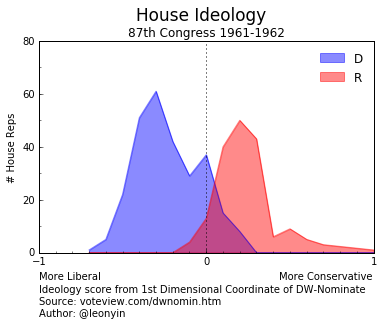We can do this for many congresses, and use the images to generate a nice gif.
For this visualization, I chose to begin at the 87th congress, while JFK was in office.

In :
def make_gif(congress, congress_start, congress_end):
'''
Saves a png for each congress into the figs subdirectory.
Uses ImageIO to combine images into a gif.
Deletes all png files in directory.
'''
for i in range(congress_start, congress_end):
plot_polarity(i, chamber=congress, how='area')

filenames = glob.glob('figs/{}/*.png'.format(congress))
images = []

for filename in filenames:
os.remove(filename)

kwargs = { 'duration': .23 }
imageio.mimsave('figs/{}/movie.gif'.format(congress),
images,  **kwargs)

In :
make_gif(congress='senate',
congress_start=87,
congress_end=114)

/Users/leonyin/anaconda/lib/python3.5/site-packages/ipykernel/__main__.py:29: SettingWithCopyWarning:
A value is trying to be set on a copy of a slice from a DataFrame.
Try using .loc[row_indexer,col_indexer] = value instead

See the caveats in the documentation: http://pandas.pydata.org/pandas-docs/stable/indexing.html#indexing-view-versus-copy
/Users/leonyin/anaconda/lib/python3.5/site-packages/ipykernel/__main__.py:31: SettingWithCopyWarning:
A value is trying to be set on a copy of a slice from a DataFrame.
Try using .loc[row_indexer,col_indexer] = value instead

See the caveats in the documentation: http://pandas.pydata.org/pandas-docs/stable/indexing.html#indexing-view-versus-copy
/Users/leonyin/anaconda/lib/python3.5/site-packages/matplotlib/pyplot.py:524: RuntimeWarning: More than 20 figures have been opened. Figures created through the pyplot interface (matplotlib.pyplot.figure) are retained until explicitly closed and may consume too much memory. (To control this warning, see the rcParam figure.max_open_warning).
max_open_warning, RuntimeWarning)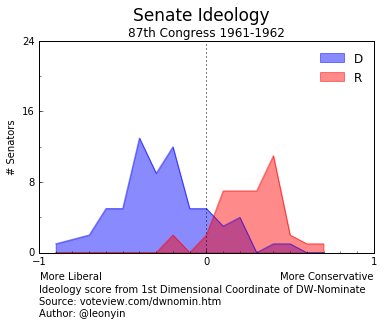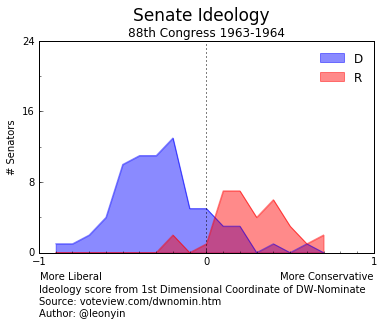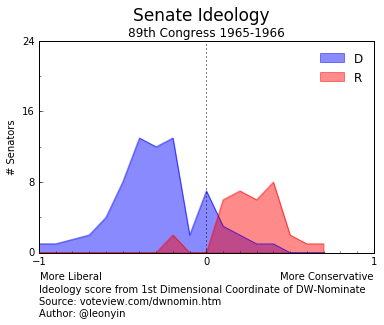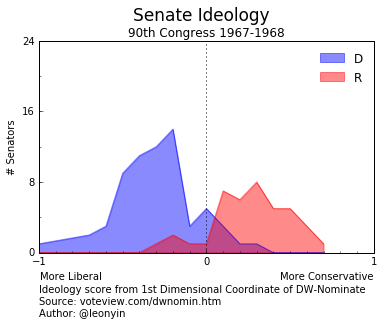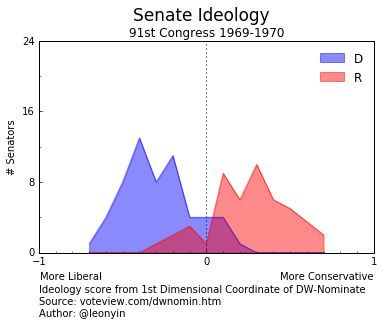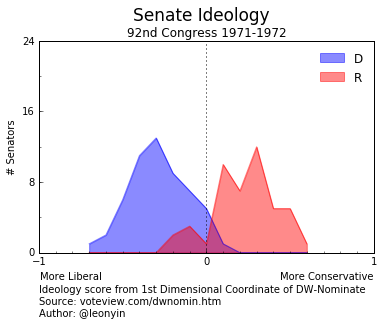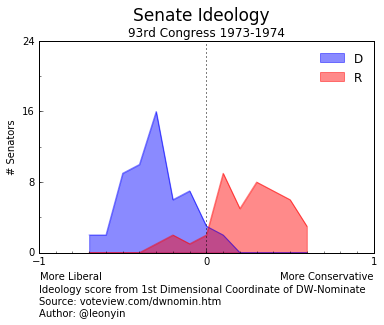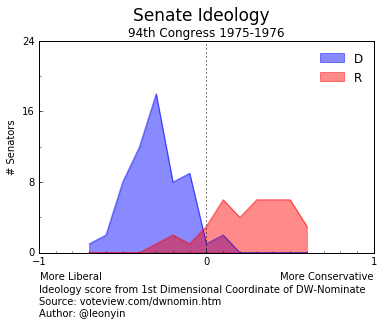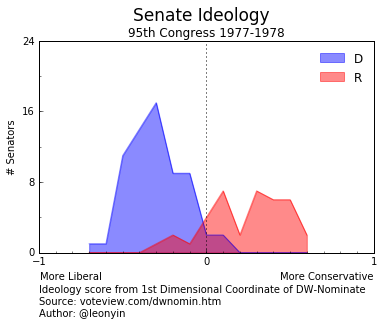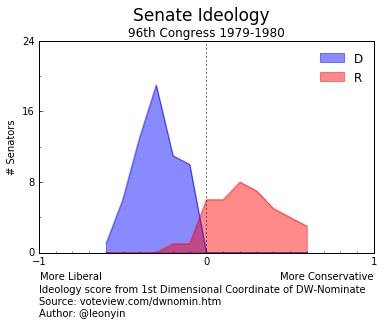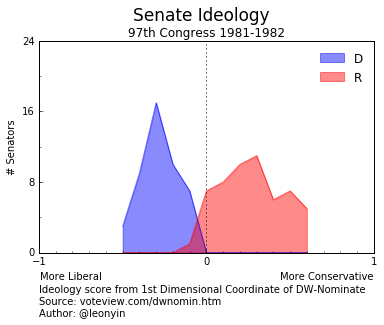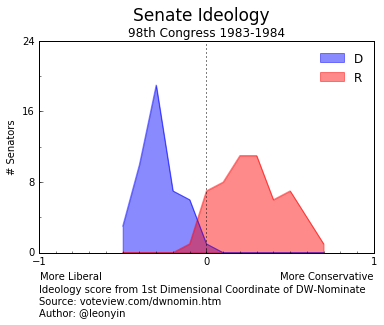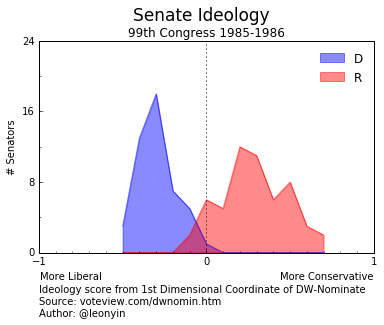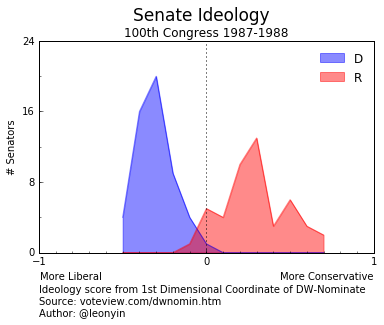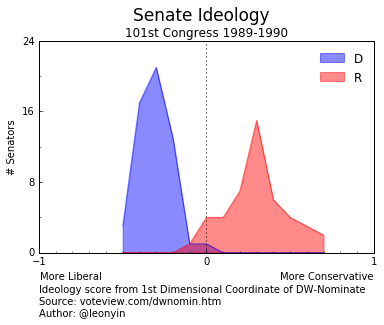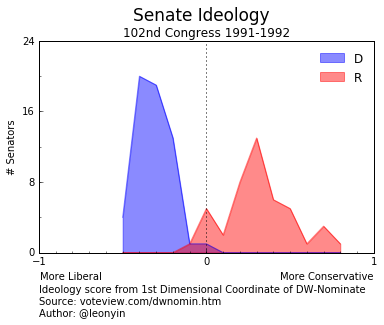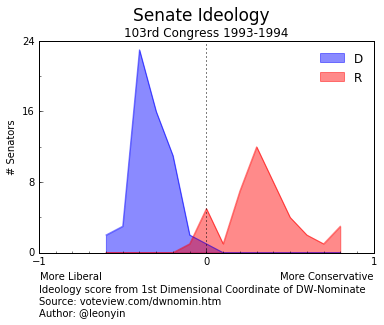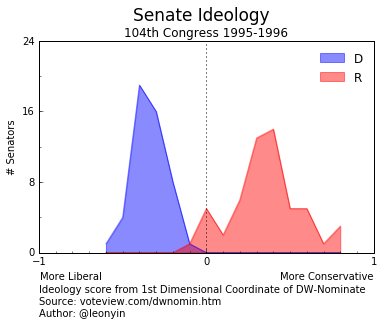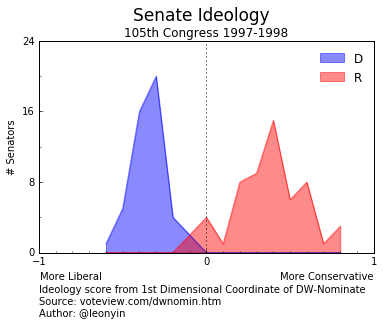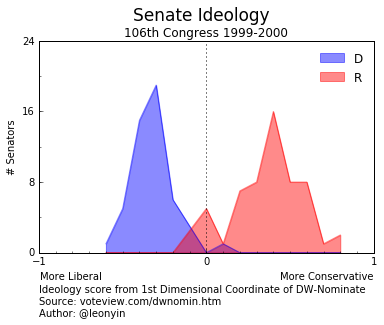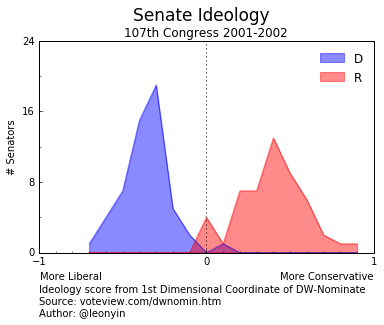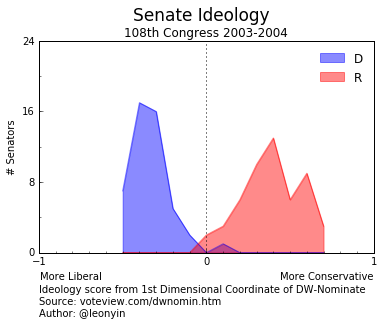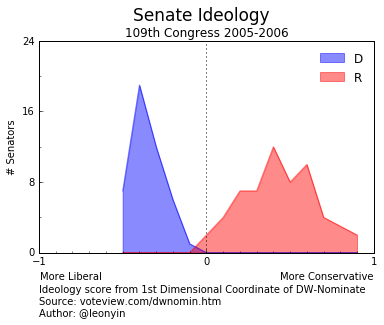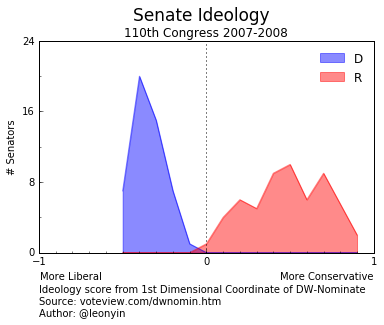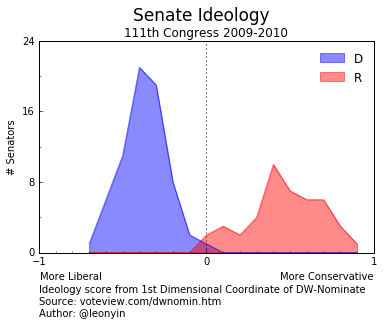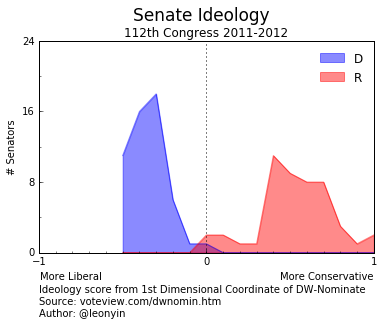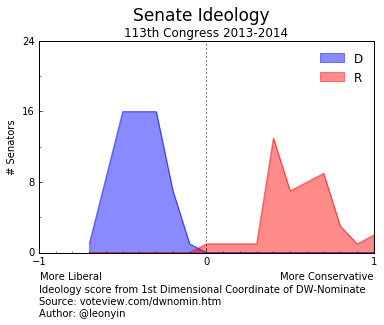Here's the finished product:GIF hosted on Github.

Notice that values on the liberal spectrum are almost never less than -0.7.
Also notice the gradual polarization between the two parties.

This is the beginning of what we're going to use the Nominate dataset for.
In the next notebook, we'll examine how we can extend the use of the Nominate dataset to the Propublica Congress API.